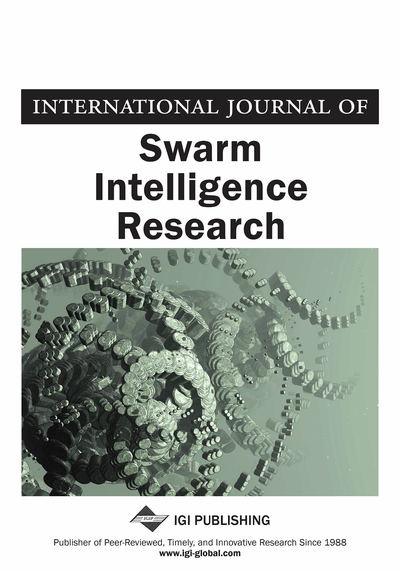# A Quantum Particle Swarm Optimization Algorithm Based on Self-Updating Mechanism

Shuyue Wu (School of Information Science & Engineering, Hunan International Economics University, Changsha, China)
DOI: 10.4018/IJSIR.2018010101

## Abstract

The living mechanism has limited life in nature; it will age and die with time. This article describes that during the progressive process, the aging mechanism is very important to keep a swarm diverse. In the quantum behavior particle swarm (QPSO) algorithm, the particles are aged and the algorithm is prematurely convergent, the self-renewal mechanism of life is introduced into QPSO algorithm, and a leading particle and challengers are introduced. When the population particles are aged and the leading power of leading particle is exhausted, a challenger particle becomes the new leader particle through the competition update mechanism, group evolution is completed and the group diversity is maintained, and the global convergence of the algorithm is proven. Next in the article, twelve Clement2009 benchmark functions are used in the experimental test, both the comparison and analysis of results of the proposed method and classical improved QPSO algorithms are given, and the simulation results show strong global finding ability of the proposed algorithm. Especially in the seven multi-model test functions, the comprehensive performance is optimal.
Article Preview

## 1. Introduction

The Particle Swarm Optimization (PSO) was proposed by Kennedy et al in the breeding behavior of simulated birds and fish in 1995 (Kennedy et al.,1995). In the evolution of the algorithm, the group shares the optimal position information (A. Manju, et al.,2014). Under the guidance of the swarm optimal position information and its own optimal information, the self-speed and position are updated by searching the multi-dimensional solution space (Zhang et al.,2016; Wu et al.,2016), and the candidate space solution is continuously followed and compared (Hao et al., 2016). And finally, the optimal solution or local optimal solution of the problem are found. In particle swarm algorithm, there are the characteristics of simple evolutionary equation, good searching ability and fast convergence speed. Particle swarm algorithm has been successfully applied in many aspects since it has been put forward. But the PSO algorithm itself is not a global optimization algorithm (Van Den Bergh, 2001), many scholars have done a lot of research work (Fang et al., 2010), they also put forward some improvement methods, and some improvement effects have been achieved (Chen et al., 2013; Campos et al., 2014). On the basis of deeply studying the evolution process of social intelligent groups, Sun et al. analyzed the mechanism of particle swarm optimization algorithm, the quantum theory was introduced to PSO algorithm, and they proposed a quantum search algorithm with global search ability (Quantum-behaved Particle Swarm Optimization, QPSO) (Sun et al., 2004; Sun et al., 2012). In QPSO algorithm, there are the characteristics of simple calculation, easy programming, less control parameters, and it has attracted the attention and research of many scholars in the related fields at home and abroad. The average value is calculated by QPSO optimal algorithms, Xi et al. introduced the nonlinear weight coefficient according to the merits of the particle, and the optimization ability of the algorithm was improved (Xi et al., 2008). Sun et al. gave the particle behavior analysis and parameter selection method of QPSO algorithm (Sun et al.,2012). In the QPSO algorithm, the mutation operator is introduced to improve the global search ability of the algorithm (Fang et al.,2009). At the same time, the QPSO algorithm is also applied to many practical problems. Omkar et al. applied the QPSO algorithm to the multi-objective optimization problem of combinatorial structure (Omkar et al.,2009). Indiral et al. applied the QPSO algorithm to association rule mining (Indiral et al., 2014), At the same time, the algorithm also has been applied in the portfolio selection problem (Farzi et al., 2013).

## Complete Article List

Search this Journal:
Reset
Open Access Articles: Forthcoming
Volume 11: 4 Issues (2020): Forthcoming, Available for Pre-Order
Volume 10: 4 Issues (2019): 3 Released, 1 Forthcoming
Volume 9: 4 Issues (2018)
Volume 8: 4 Issues (2017)
Volume 7: 4 Issues (2016)
Volume 6: 4 Issues (2015)
Volume 5: 4 Issues (2014)
Volume 4: 4 Issues (2013)
Volume 3: 4 Issues (2012)
Volume 2: 4 Issues (2011)
Volume 1: 4 Issues (2010)
View Complete Journal Contents Listing